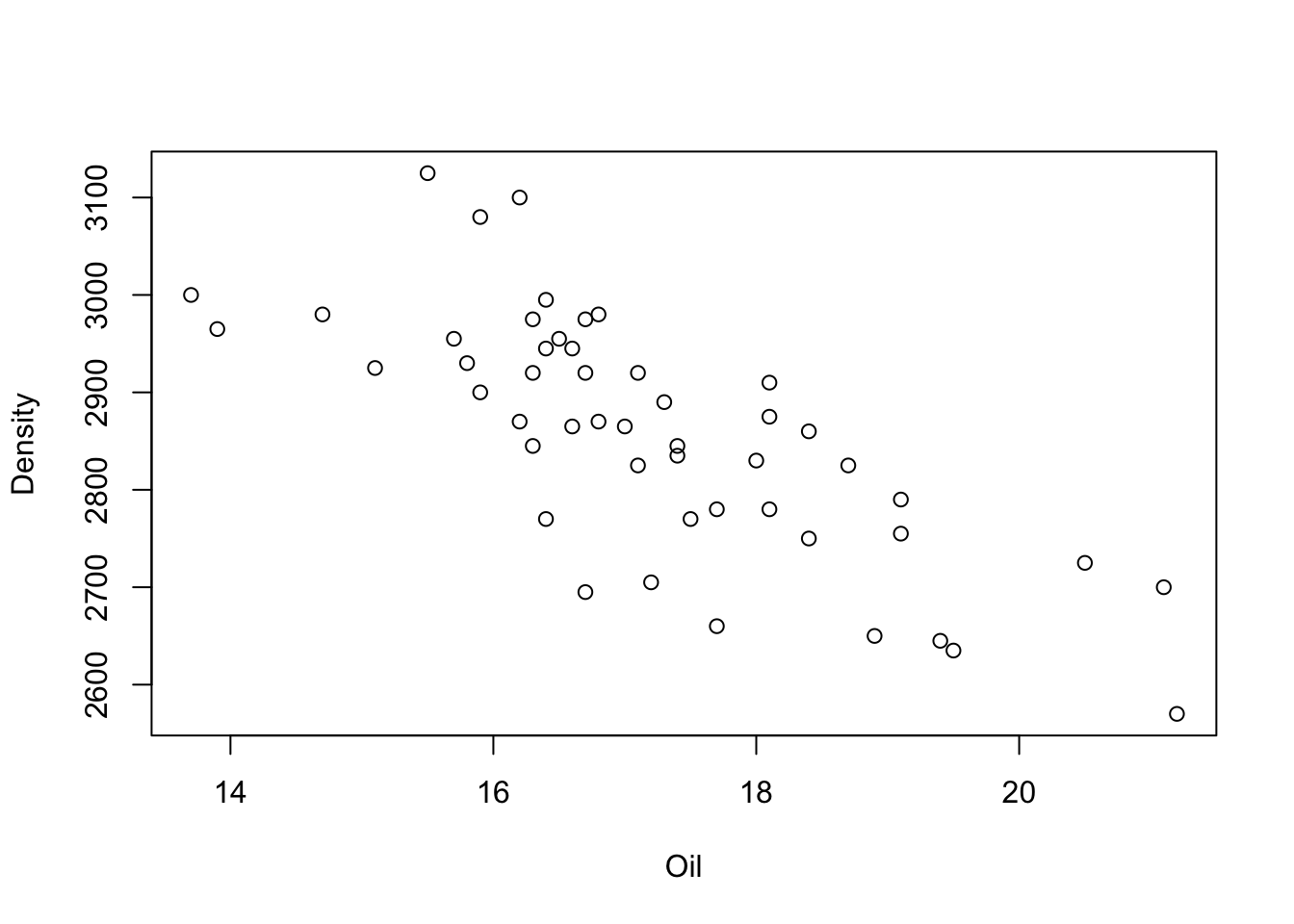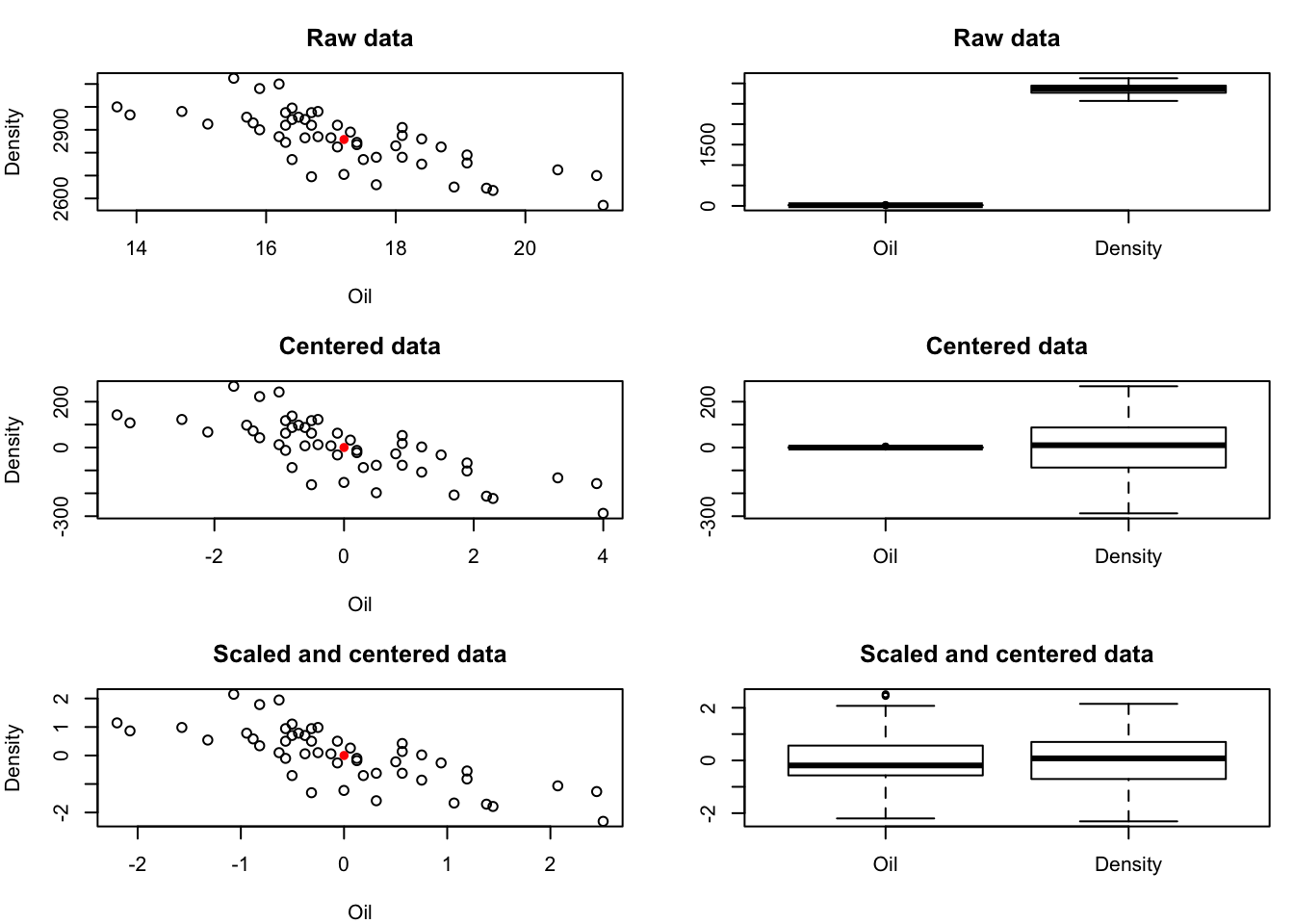PCA starts with data. In this section we analyse the food texture data set. This open source data set is available here and describes texture measurements of a pastry-type food.

food <- read.csv("https://userpage.fu-berlin.de/soga/300/30100_data_sets/food-texture.csv")
str(food)
## 'data.frame':    50 obs. of  6 variables:
##  $X : Factor w/ 50 levels "B110","B136",..: 1 2 3 4 5 6 7 8 9 10 ... ##$ Oil     : num  16.5 17.7 16.2 16.7 16.3 19.1 18.4 17.5 15.7 16.4 ...
##  $Density : int 2955 2660 2870 2920 2975 2790 2750 2770 2955 2945 ... ##$ Crispy  : int  10 14 12 10 11 13 13 10 11 11 ...
##  $Fracture: int 23 9 17 31 26 16 17 26 23 24 ... ##$ Hardness: int  97 139 143 95 143 189 114 63 123 132 ...

The data set consists of 50 rows (observations) and 6 columns (features/variables). The features are:

• Oil: percentage oil in the pastry
• Density: the product’s density (the higher the number, the more dense the product)
• Crispy: a crispiness measurement, on a scale from 7 to 15, with 15 being more crispy.
• Fracture: the angle, in degrees, through which the pasty can be slowly bent before it fractures.
• Hardness: a sharp point is used to measure the amount of force required before breakage occurs.

For the sake of comprehensibility we start working with a reduced, two-dimensional toy data set, by extracting the columns Oil and Density. In a subsequent section we return to the full data set for our analyses.

pca.toy <- food[, c('Oil', 'Density')]

We start with an exploratory data analysis and examine a scatter plot.

plot(pca.toy)#### Scale the data

The scatter plot above indicates a relationship between the feature Oil and the feature Density. Note that the variables are not on the same scale. In general, the results of PCA depend on the scale of the variables. Therefore, each variable is typically centered and scaled to have a mean of zero and standard deviation of one. In certain settings, however, the variables are measured in the same units, and one may skip the standardization.

For the sake of comprehensibility we write two simple functions in R and visualize the effects of each pre-processing step. The goal is to center each column to zero mean and then scale it to have unit variance. For further usage we assign a proper variable name (e.g. pca.toy.data) to pre-processed data set.

# Function for centering a vector
center <- function(v){v-mean(v)}
# Function for scaling a vector
scale <- function(v){v/sd(v)}

## save helper functions for later usage
save(center, scale, file = 'helper_functions_30300.RData')

# Apply use defined function on each column of the data set
pca.toy.centered <- apply(pca.toy, MARGIN = 2, FUN = center)
pca.toy.scaled <- apply(pca.toy.centered , MARGIN = 2, FUN = scale)

### Plotting ###
par(mfrow = c(3,2), mar = c(4,4,3,1))
###############
# scatterplot 1
plot(pca.toy, main = 'Raw data')
# calulate mean for visualization
data.mean <- apply(pca.toy, 2, mean)
points(data.mean, data.mean, col='red', pch=16) # mark mean
# boxplot 1
boxplot(pca.toy, main = 'Raw data')
###############

# scatterplot 2
plot(pca.toy.centered , main = 'Centered data')
# calulate mean for visualization
data.mean <- apply(pca.toy.centered, 2, mean)
points(data.mean, data.mean, col='red', pch=16) # mark mean
# boxplot 2
boxplot(pca.toy.centered , main = 'Centered data')
###############

# scatterplot 3
plot(pca.toy.scaled, main = 'Scaled and centered data')
# calulate mean for visualization
data.mean <- apply(pca.toy.scaled, 2, mean)
points(data.mean, data.mean, col='red', pch=16) # mark mean
# boxplot 3
boxplot(pca.toy.scaled, main = 'Scaled and centered data')# assign propper variable name to pre-processed data set
pca.toy.data <- pca.toy.scaled

# save for later usage
save(pca.toy.data, file = 'pca_food_toy_30300.RData')